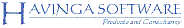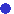Havinga Software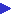ThreeDimSimFeatures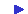Examples...Car break thruHourglassSimple carJumping BipedMotor controlMini BipedTippe-topEnv. mappingBilliard play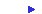Satellite orbitingScreenshotHow it worksSystem modelingQuick tutorialDownloadSupport

# ThreeDimSim: Satellite orbiting

This example shows how other physical phenomena can be simulated by using the capability of ThreeDimSim to include user defined forces in simulation. In this case the sphere in the middle represents the earth. Furthermore two objects are circling around the earth at 36000 km (yellow trace) and at 3600 km height (red trace). As you can see the yellow trace is indeed orbiting as fast as the earth is turning around, as one would expect at this geo-stationary orbit. The lower orbiting object is moving much faster.The attracting force keeping the objects in orbit is defined by Newton's law of gravitation:

Fab = G * ma* mb / rab2.

In script this force is defined in a function f_satellite, as follows:

```function fn_satellite()
{
return G * earth.mass * satellite.mass / sqr(norm(satellite.position));
}```

Then a force is defined acting between earth and satellite, using the previous defined function:

```var f_satellite = Force(earth, {0,0,0}, satellite, {0,0,0});
f_satellite.force = fn_satellite;```

Now, at each simulation step, the force will evaluate its assigned function to calculate the force to be applied between its two assigned objects.

The principle of assigning functions to properties can be utilized on many other properties as well.